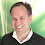## December 9, 2013

### MathML: A Test

This blog post is to test how well MathLM works with browsers and with Blogger

This expression: ${\int }_{-1}^{1}\sqrt{1-{x}^{2}}dx=\frac{\pi }{2}$ is pretty cool to have inline.

${S}_{i+1}={t}_{i}\left({S}_{i}\right)$

The MathML code was generated with the editor at

http://www1.chapman.edu/~jipsen/mathml/asciimatheditor/

1.2.3.